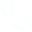# [information Security] Principle of Rsa Asymmetric Encryption Algorithm

[information Security] Principle of Rsa Asymmetric Encryption Algorithm

[Information Security] Principle of RSA asymmetric encryption algorithm (detailed explanation and C++ code implementation)
1. RSA asymmetric encryption
(1) Select two prime numbers p and q, calculate n=p*q and Euler function φ(n)=(p-1)(q-1), select integer e, make gcd (φ(n), e )=1 (that is, φ(n) and e are co-prime), 1(2) Calculate the inverse of e d=e-1mod φ(n) (ie ed = 1 mod φ(n));
(3) Obtain public key Kpub = {e, n}, private key Kpri = {d, n} (public public key Kpub , secret private key Kpri );
(4) Encryption (using public key Kpub ): for plaintext m(5) Decryption (using the private key Kpri ): for the ciphertext c, the plaintext m=cd mod n
2. RSA encryption and decryption example

3. Code implementation ( c++ )
#include
using namespace std;

// greatest common factor
int maxCommonDivisor ( int a, int b)
{
int temp = a;
if (a < b)
{
a = b;
b = temp;
}

while( a % b)
{
temp = b;
b = a % b;
a = temp;
}
return b;
}

// least common multiple
int leastCommonMultiple ( int a, int b)
{
int macDivisor = maxCommonDivisor ( a, b);
return a / macDivisor * b;
}

// Calculate input ^ rate mod y
int multiMod(int input, int rate, int y)
{
int start = 1;
int arr;
arr = 1;
arr = input;
int step = 1;
int result = 1;

while(rate)
{
if (step == 1)
{
arr[step] = input;
}
else
{
arr[step] = arr[step - 1] * arr[step - 1];
arr[step] %= y;
}
if(rate&1)
{
result *= arr[step];
result %= y;
}
step ++;
rate = rate >> 1;
}

return result;
}

int main()
{
int input;
int p, q;
int N, L, E, D;

while(cin >> p >> q >> input >> E)
{
N = p * q;
//最小公倍数
L = leastCommonMultiple(p - 1, q - 1);
//E * D mod L = 1
int X = 1;
while((X * L + 1) % E)
{
X ++;
}

D = (X * L + 1) / E;
cout <<"N = " << N << " L = " << L << " E = " << E << " D = " << D << " X = " << X << endl ;
// encryption process
int code = multiMod ( input, E, N);
// decryption process
int deCode = multiMod ( code, D, N);
cout<< "code = " << code << " deCode = " << deCode << endl;
}
}

## Related Articles

• ### A detailed explanation of Hadoop core architecture HDFS

Knowledge Base Team

• ### What Does IOT Mean

Knowledge Base Team

• ### 6 Optional Technologies for Data Storage

Knowledge Base Team

• ### What Is Blockchain Technology

Knowledge Base Team

## Explore More Special Offers

1. ### Short Message Service(SMS) & Mail Service

50,000 email package starts as low as USD 1.99, 120 short messages start at only USD 1.00Contact Us